﻿ 基于支持向量的最近邻文本分类方法
«上一篇文章快速检索 高级检索

 智能系统学报2018, Vol. 13Issue (5): 799-807  DOI: 10.11992/tis.2017110070

### 引用本文GULNAZ Alimjan, HURXIDA Jumahun, SUN Tieli, et al. The nearest neighbor text classification method based on support vector[J]. CAAI Transactions on Intelligent Systems, 2018, 13(5): 799-807. DOI: 10.11992/tis.201711007.### 文章历史

1. 伊犁师范学院 电子与信息工程学院，新疆 伊宁 835000;
2. 东北师范大学 计算机科学与技术学院，吉林 长春 130117;
3. 东北师范大学 地理科学学院，吉林 长春 130024

The nearest neighbor text classification method based on support vector
GULNAZ Alimjan1,2,3, HURXIDA Jumahun1, SUN Tieli2, LIANG Yi1
1. Department of Electronics and Information Engineering, Yili Normal University, Yining 835000, China;
2. School of Information Science and Technology, Northeast Normal University, Changchun 130117, China;
3. Department of Geographical Science, Northeast Normal University, Changchun 130024, China
Abstract: Text categorization automatically assigns a set of predefined categories or topics to a document. In text classification, the representation of the document has a great influence on the learning performance of the learning machine. The aim is to achieve Kazakh text classification, according to Kazakh grammar rules, the stemming of Kazakh texts is designed to complete the preprocessing of Kazakh text. A sample distance formula based on the latest support vector machine (SVM) is proposed to avoid the selection of k-parameters. The Kazakh texts are classified by special combination of SVM and KNN classification algorithms (SV-NN) . Combining the corpus of Kazakh text corpora constructed by himself, text categorization simulation experiments were conducted. Numerical experiments showed the effectiveness of the proposed algorithm and confirmed the theoretical results.
Key words: stemming    preprocessing    support vector machines    text categorization    classification accuracy

1 文本特征提取 1.1 文本预处理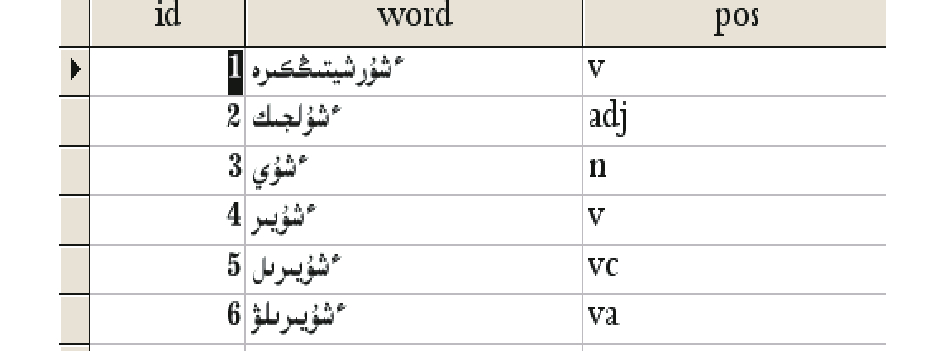Download: 图 1 哈萨克语词干 Fig. 1 Kazakh text stem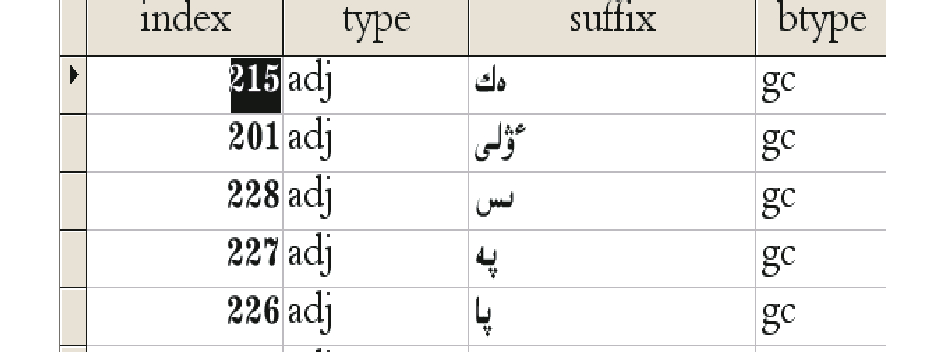Download: 图 2 哈萨克语附加成分 Fig. 2 Additional components in Kazakh text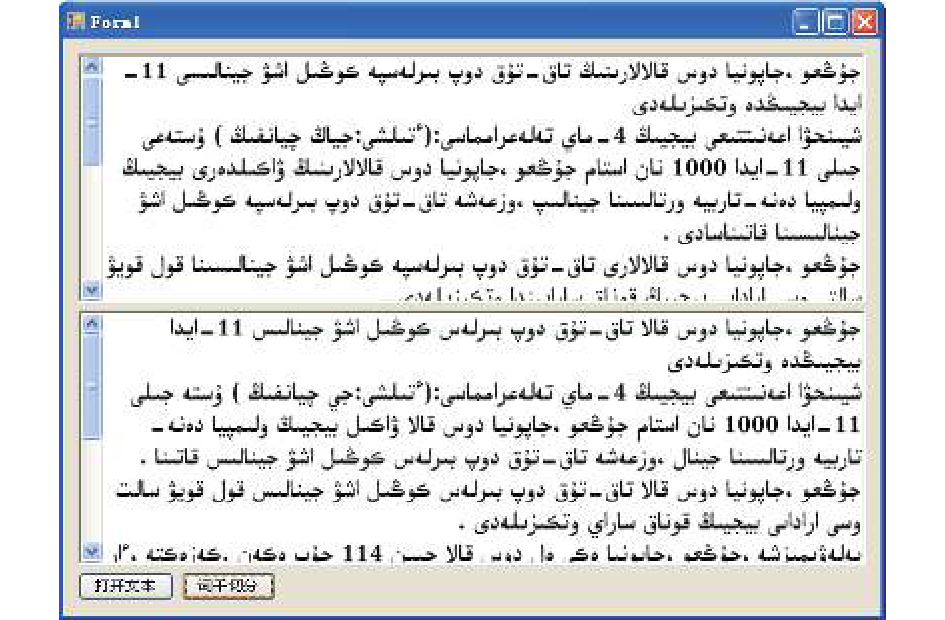Download: 图 3 哈萨克语文本词干切分结果示例 Fig. 3 Example segmentation results of the Kazakh text stem
1.2 特征处理

 ${\rm DFR}\left( {{{t}},{C_i}} \right) = \frac{{\left( {N - {n_i}} \right) \times {\rm D{F}_i}}}{{{n_i} \times {\rm DF}_i'}}$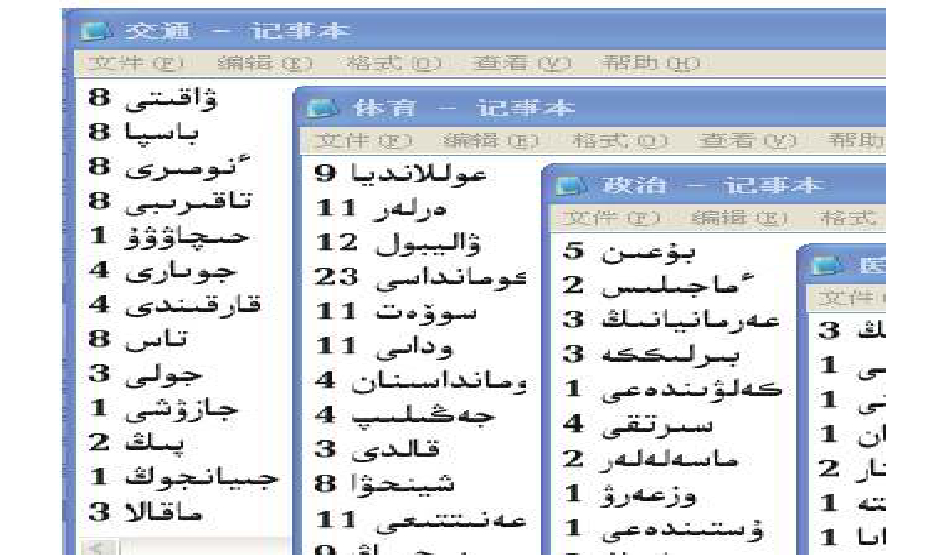Download: 图 4 词频统计结果 Fig. 4 Term frequency statistical result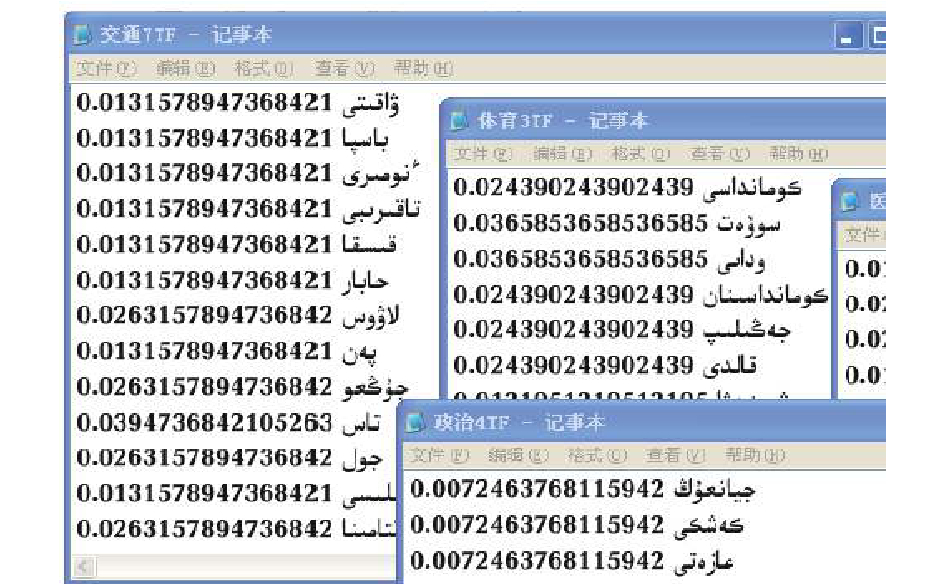Download: 图 5 词权重计算结果 Fig. 5 Term weight computed result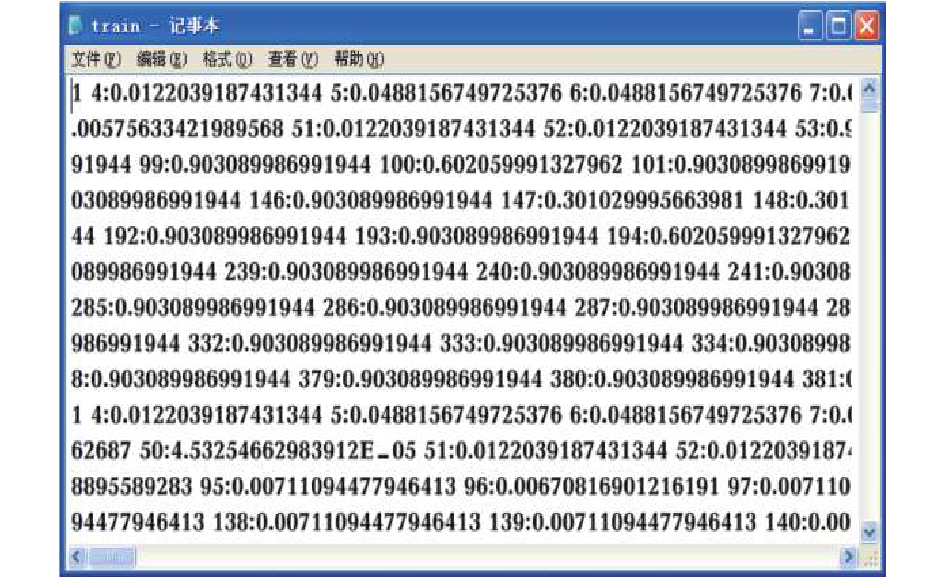Download: 图 6 文本向量文件 Fig. 6 Text vector files
2 SVM与KNN方法 2.1 SVM方法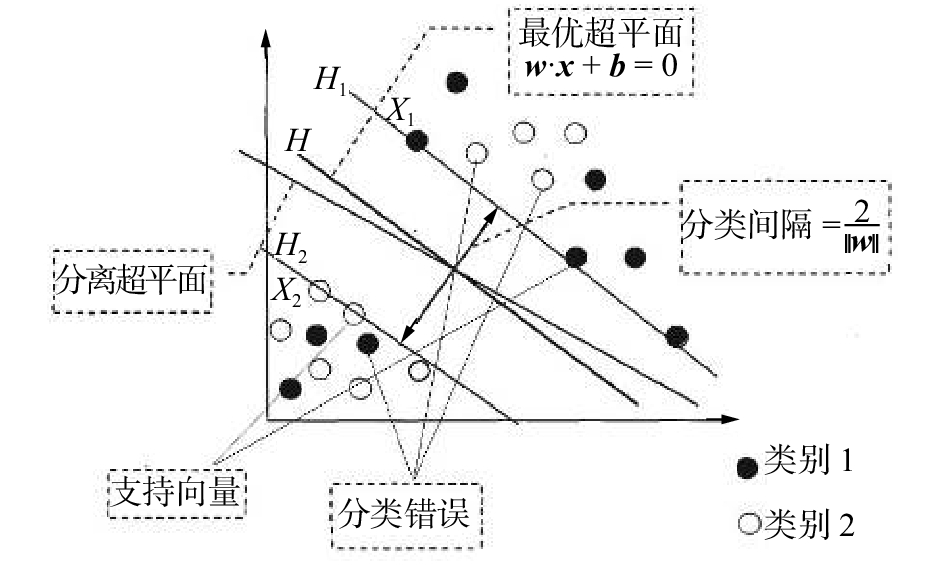Download: 图 7 SVM 分类原理示意图 Fig. 7 SVM classification schematic diagram

1)线性可分

 $\begin{array}{c}S = \left\{ {\left( {{x_i} , {y_i}} \right),i = 1,2, \cdots ,r} \right\},{x_i} \in {{\bf R}^n},{y_i} \in \left\{ { + 1, - 1} \right\}\end{array}$ (1)
 {\begin{aligned}\min \varphi \left( { \omega} \right) = \displaystyle\frac{1}{2}{\left\| { \omega} \right\|^2},{\rm s.t.}{y_i}\left[ {{ \omega} {x_i} + b} \right] - 1\geqslant 0,\;\;i = 1,2, \cdots ,n\end{aligned}} (2)

 $f\left( x \right) = {\rm sign}\left( {\sum\limits_{i = 1}^n {{a_i}} {y_i}\left( {{x_i} \cdot x} \right) + b} \right)$ (3)

2)非线性可分

SVM通过运用合适的非线性映射，如 $\varphi :{x_i} \to \varphi \left( {{x_i}} \right)$ 把分类问题原训练样本点转变(映射)到新的地方(新特征空间)，使得原样本在这新特征空间(目标高维空间)中能够线性可分，然后在这新的映射空间中利用线性可分问题求解原理求出最终的最优分类面。

 {\begin{aligned}\min \varphi \left( {\omega ,\xi } \right) = \displaystyle\frac{1}{2}{\left\| \omega \right\|^2} + C\displaystyle\sum\limits_{i = 1}^n {{\xi _i}} ,\,\,{\rm s.t.}{y_i}\left[ {\omega {x_i} + b} \right] - 1 + {\xi _i} \geqslant 0\end{aligned}} (4)

SVM通过引入一个核函数K(xi·x)，将原低维的分类问题空间映射到高维的新问题空间中，使核函数代替 ${{\omega}} \cdot \varphi \left( x \right)$ 内积运算，这个高维空间就称为Hilbert空间。引入核函数以后的最优分类函数为

 $f\left( x \right) = {\rm sign}\left( {\sum\limits_{i = 1}^n {{a_i}} {y_i}K\left( {{x_i} \cdot x} \right) + b} \right)$ (5)
2.2 KNN方法

KNN(K nearest neighbor)分类法是基于实例的学习算法，它需要所有的训练样本都参与分类。在分类阶段，利用欧氏距离公式，将每个测试样本与和它邻近的k个训练样本进行比较，然后将测试样本归属到票数最多的那一类里。KNN算法是根据测试样本最近的k个样本点的类别信息来对该测试样本类型进行判别，所以k值的选取非常重要。k值若太小，测试样本特征不能充分体现；k值若太大，与测试样本并不相似的个别样本也可能被包含进来，这样反而对分类不利。KNN算法在分类决策上只凭k个最邻近样本类别确定待分样本的所属类。目前，对于k值的选取还没有一个全局最优的筛选方法，这也是KNN方法的弊端，具体操作时，根据先验知识先给出一个初始值，然后需要根据仿真分类实验结果重新调整，调整k值的操作有时一直到分类结果满足用户需求为止。该方法原理可由式(7)表示：

 $y\left( {{d_i}} \right) = \arg {\max _k}\sum\limits_{{x_j} \in {\rm KNN}} y \left( {{x_j},{c_k}} \right)$ (6)

 $y\left( {{d_i}} \right) = \arg {\max _k}\sum\limits_{{x_j} \in {\rm KNN}} {\rm Sim} \left( {{d_i},{x_j}} \right)y\left( {{x_j},{c_k}} \right)$ (7)

xjci时， $y\left( {{x_j},{c_i}} \right) = 1$ ；当xj $\notin$ ci时， $y\left( {{x_j},{c_i}} \right) = 0$ ${\rm Sim}\left( {{d_i},{x_j}} \right)$ 是测试样本di和它最近邻xj之间的余弦相似度。余弦相似度测量是由一个向量空间中两个向量之间角余弦值来定义的。式(8)说明测试样本di被归到k个最近邻类里相似性最大那个类别里。

KNN虽是简单有效的分类方法，但不能忽略以下两方面的问题：1)由于KNN需要保留分类过程中的所有相似性计算实例，从而随着训练集规模的增多，其计算量也会增加，在处理较大规模数据集分类时方法的时间复杂度会达到不可接受的程度，这是KNN方法的主要缺点；2) KNN方法分类的准确性可能受到训练数据集中特性的无关性和噪声数据的影响，若考虑这些因素其分类效果也许更好。

3 基于SV-NN的哈萨克语文本分类算法

3.1 SV-NN算法描述及流程图

SV-NN 分类算法：

Start:

{ integer i, j,k,l

i=1; j=1; k=1；// $i = 1,2, \cdots ,n;j = 1,2, \cdots ,m;$

SVM: T1→svij；//通过使用SVM定义每个类别的支持向量。

while(k<l)

{ 输入 xk

xk划入到基于 $\mathop {\min }\limits_k \left( {{\rm aver}{D_k}} \right)$ 的最近类别；

k=k+1;

}

}

End

SV-NN分类方法的工作流程如图8所示。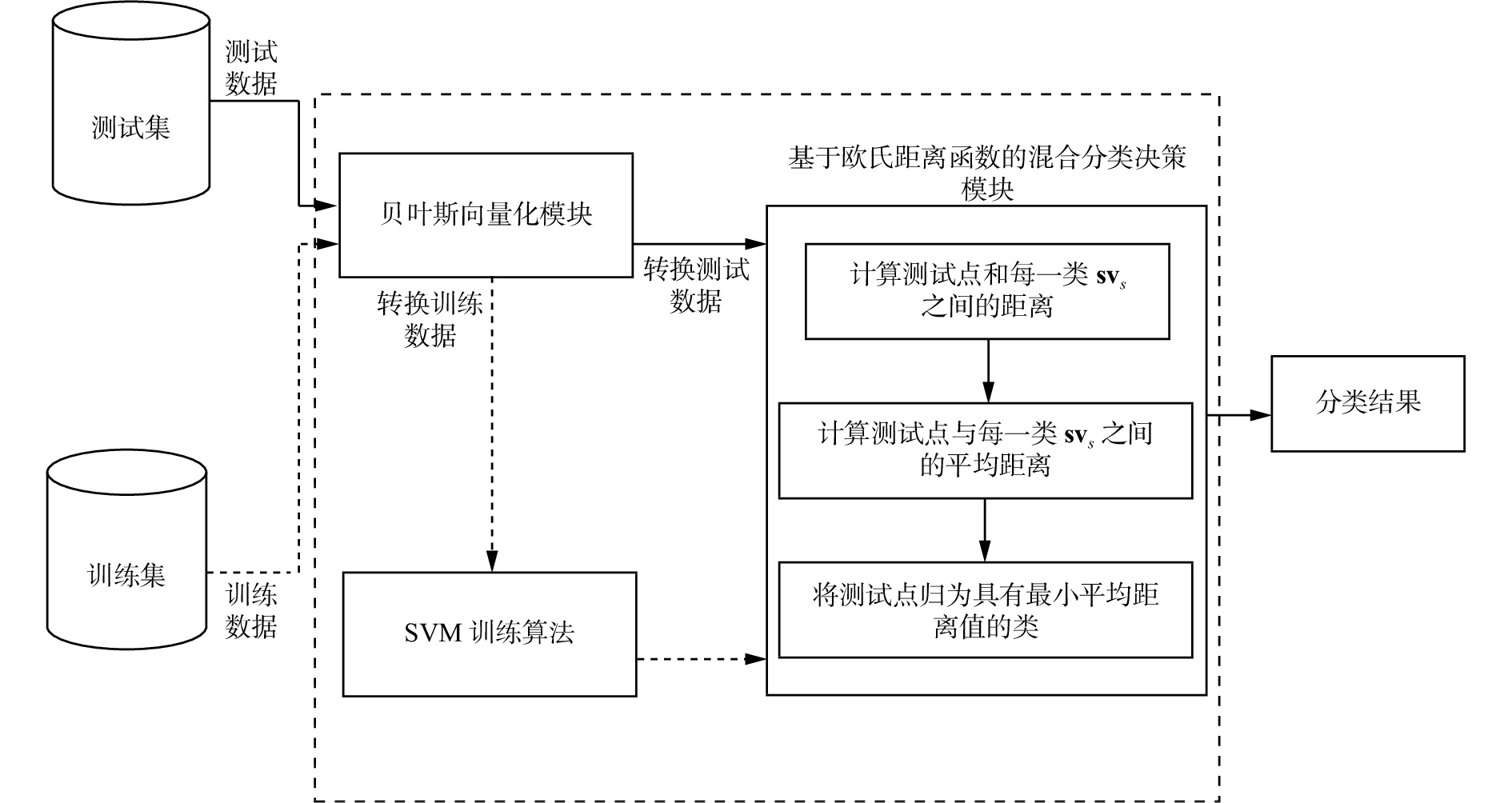Download: 图 8 SV-NN分类方法工作原理 Fig. 8 SV-NN classification approach working principle diagram
3.2 SV-NN 算法实现

1)将所有训练点映射到向量空间，并通过传统SVM确定每一个类别的支持向量。

 $\left( {\begin{array}{*{20}{c}}{{{{\bf{sv}}}_{11}}}& { {{{\bf{sv}}}_{12}}}&\cdots &{ {{{\bf{sv}}}_{1m}}}\\ { {{{\bf{sv}}}}_{21}} & {{{{\bf{sv}}}}_{22}}& \cdots & {{ {{\bf{sv}}}_{2m}}} \\ \vdots & \vdots &{\rm{}}&\vdots\\{{{{\bf{sv}}}_{n1}}}&{{{{\bf{sv}}}_{n2}}} & \cdots &{ {{{\bf{sv}}}_{nm}}}\end{array}} \right),\;\;i = 1,2, \cdots ,n;j = 1,2, \cdots ,m$ (8)

2)使用欧氏距离公式(9)计算测试样本xk与由1)生成的每一类支持向量svij之间的距离。

 $\begin{array}{c}{D_{kj}} =\displaystyle \sum\limits_{k = 1}^l {\sqrt {\left( {{{\displaystyle\sum\limits_{i = 1}^n {\left( {{x_k} - \displaystyle\sum\limits_{j = 1}^m {{{{{\bf{sv}}}}_{ij}}} } \right)} }^2}} \right)} } \end{array}$ (9)

3)使用式(10)计算测试样本xk与每一类支持向量svij之间的平均距离：

 ${\rm aver}{D_k} = \frac{{\displaystyle\sum\limits_{j = 1}^m {{D_{kj}}} }}{m},\;\;j = 1,2, \cdots ,m;k = 1,2, \cdots ,l$ (10)

4）计算最短平均距离minD，并将测试样本xk划入到最短平均距离对应的那一类中。

 $\min D = \mathop {\min }\limits_k \left( {{\rm aver}{D_k}} \right),\;\;k = 1,2, \cdots ,l$ (11)

4 实验结果与评价表 1 数据集 Tab.1 Data set表 2 SVM、KNN、SV-NN的分类精度对比 Tab.2 SVM KNN and SV–NN comparison of classification accuracy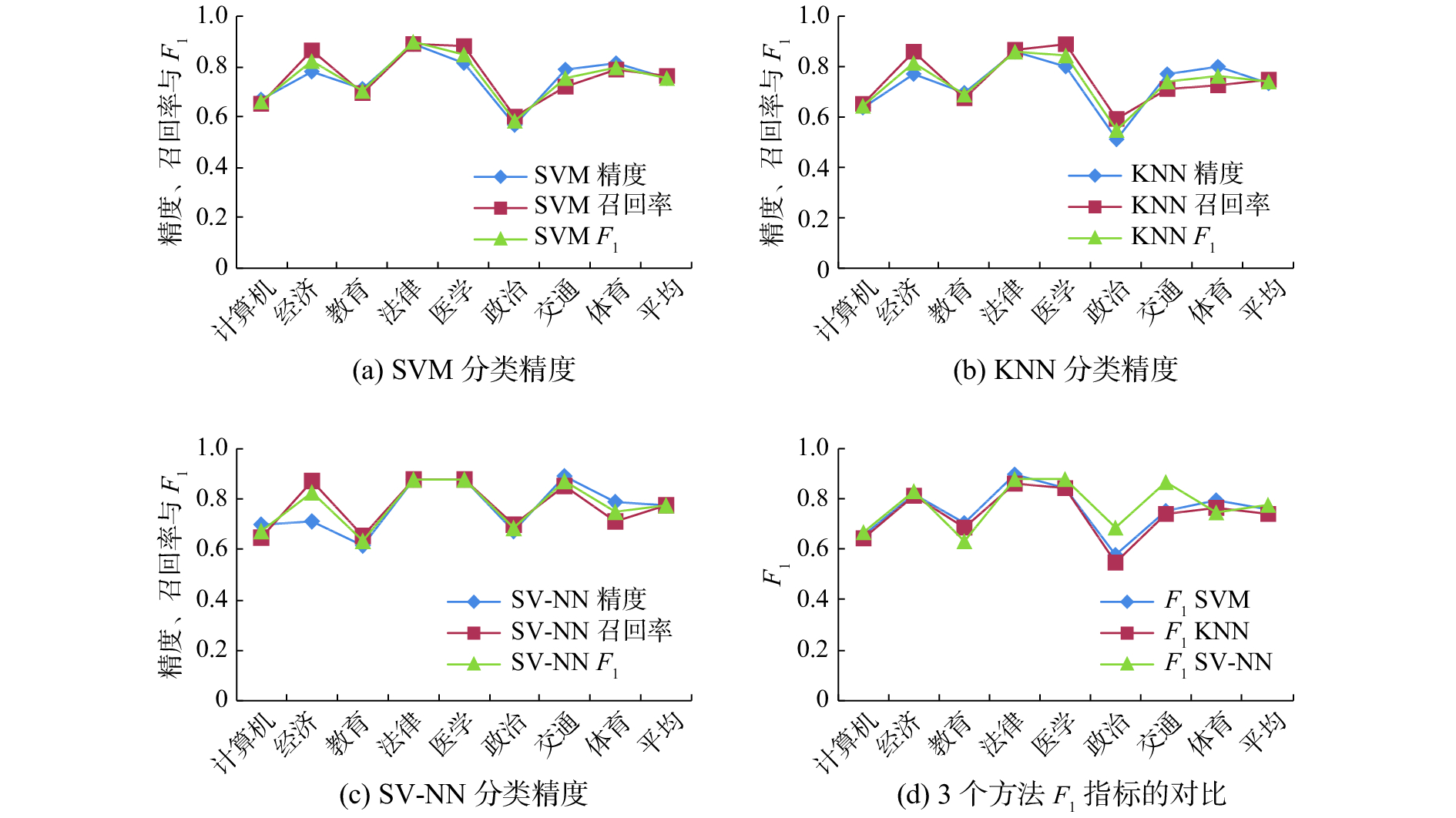Download: 图 9 分类精度的对比分析（每一类别均含175篇文档） Fig. 9 Comparative analysis of classification accuracy (each category contains 175 documents)
5 结束语

  SEBASTIANI F. Machine learning in automated text categorization[J]. ACM computing surveys, 2002, 34(1): 1-47. DOI:10.1145/505282.505283 (0)  AHMADI A, FOTOUHI M, KHALEGHI M. Intelligent classification of web pages using contextual and visual features[J]. Applied soft computing, 2011, 11(2): 1638-1647. DOI:10.1016/j.asoc.2010.05.003 (0)  MARTÍNEZ-CÁMARA E, MARTÍN-VALDIVIA M T, UREÑA-LÓPEZ L A, et al. Polarity classification for Spanish tweets using the COST corpus[J]. Journal of information science, 2015, 41(3): 263-272. DOI:10.1177/0165551514566564 (0)  PERCANNELLA G, SORRENTINO D, VENTO M. Automatic indexing of news videos through text classification techniques[M]//SINGH S, SINGH M, APTE C, et al. Pattern Recognition and Image Analysis. Berlin: Springer, 2005: 512–521. (0)  HU Rong, NAMEE B M, DELANY S J. Active learning for text classification with reusability[J]. Expert systems with applications, 2016, 45: 438-449. DOI:10.1016/j.eswa.2015.10.003 (0)  SAKURAI S, SUYAMA A. An e-mail analysis method based on text mining techniques[J]. Applied soft computing, 2005, 6(1): 62-71. DOI:10.1016/j.asoc.2004.10.007 (0)  AL-KABI M, WAHSHEH H, ALSMADI I, et al. Content-based analysis to detect Arabic web spam[J]. Journal of information science, 2012, 38(3): 284-296. DOI:10.1177/0165551512439173 (0)  ZITAR R A, MOHAMMAD A H. Spam detection using genetic assisted artificial immune system[J]. International journal of pattern recognition and artificial intelligence, 2011, 25(8): 1275-1295. DOI:10.1142/S0218001411009123 (0)  MOHAMMAD A H, ZITAR R A. Application of genetic optimized artificial immune system and neural networks in spam detection[J]. Applied soft computing, 2011, 11(4): 3827-3845. DOI:10.1016/j.asoc.2011.02.021 (0)  MAO Ming, PENG Yefei, SORING M. Ontology mapping: As a binary classification problem[J]. Concurrency and computation: practice and experience, 2011, 23(9): 1010-1025. DOI:10.1002/cpe.v23.9 (0)  YANG Yiming, SLATTERY S, GHANI R. A study of approaches to hypertext categorization[J]. Journal of intelligent information systems, 2002, 18(2/3): 219-241. DOI:10.1023/A:1013685612819 (0)  REN Fuji, LI Chao. Hybrid Chinese text classification approach using general knowledge from Baidu Baike[J]. IEEJ transactions on electrical and electronic engineering, 2016, 11(4): 488-498. DOI:10.1002/tee.2016.11.issue-4 (0)  DUWAIRI R, EL-ORFALI M. A study of the effects of preprocessing strategies on sentiment analysis for Arabic text[J]. Journal of information science, 2014, 40(4): 501-513. DOI:10.1177/0165551514534143 (0)  张冬梅. 文本情感分类及观点摘要关键问题研究[D]. 济南: 山东大学, 2012. ZHANG Dongmei. Research on key problems in text sentiment classification and opinion summarization[D]. Ji’nan: Shandong University, 2012. (0)  杨杰明. 文本分类中文本表示模型和特征选择算法研究[D]. 长春: 吉林大学, 2013. YANG Jieming. The research of text representation and feature selection in text categorization[D]. Changchun: Jilin University, 2013. (0)  张晓娜. CNNIC发布第37次中国互联网络发展状况统计报告[N]. 民主与法制时报, 2016-01-23(001). (0)  SYIAM M M, FAYED Z T, HABIB M B. An intelligent system for Arabic text categorization[J]. International journal of cooperative information systems, 2006, 6(1): 1-19. (0)  DUWAIRI R, AL-REFAI M, KHASAWNEH N. Stemming versus light stemming as feature selection techniques for Arabic text categorization[C]//Proceedings of the 4th International Conference on Innovations in Information Technology. Dubai, 2007: 446–450. (0)  贺慧, 王俊义. 主动支持向量机的研究及其在蒙文文本分类中的应用[J]. 内蒙古大学学报: 自然科学版, 2006, 37(5): 560-563. HE Hui, WANG Junyi. Study of active learning support vector machine and its application on mongolian text classification[J]. Acta scientiarum naturalium universitatis neimongol, 2006, 37(5): 560-563. (0)  ADELEKE A O, SAMSUDIN N A, MUSTAPHA A, et al. Comparative analysis of text classification algorithms for automated labelling of quranic verses[J]. International journal on advanced science engineering information technology, 2017, 7(4): 1419-1427. DOI:10.18517/ijaseit.7.4.2198 (0)  MOHAMMAD A H, ALWADA N T, AL-MOMANI O. Arabic text categorization using support vector machine, naïve bayes and neural network[J]. GSTF journal on computing, 2016, 5(1): 1-8. (0)  古丽娜孜·艾力木江, 孙铁利, 伊力亚尔·加尔木哈, 等. 一种基于主动学习支持向量机哈萨克文文本分类方法[J]. 智能系统学报, 2011, 6(3): 261-267. GU Linazi Ai Limujiang, SUN Tieli, Yi Liyaer Jia Ermuhamaiti, et al. An approach to the text categorization of the Kazakh language based on an active learning support vector machine[J]. CAAI transactions on intelligent systems, 2011, 6(3): 261-267. DOI:10.3969/j.issn.1673-4785.2011.03.011 (0)  古丽娜孜·艾力木江, 孙铁利, 乎西旦·居马洪, 等. 一种基于改进KNN的哈萨克语文本分类[J]. 东北师大学报: 自然科学版, 2014, 46(2): 63-68. GU Linazi Ai Limujiang, SUNTieli, HU Xidan Ju Mahong, et al. Textcategorization of kazakh text based on improved KNN[J]. Journal of northeast normal university: natural science edition, 2014, 46(2): 63-68. (0)  古丽娜孜·艾力木江, 孙铁利, 乎西旦·居马洪, 等. 一种基于SVM-修正KNN算法的哈萨克语文本分类[J]. 西北师范大学学报: 自然科学版, 2014, 50(3): 48-53. GU Linazi Ai Limujiang, SUN Tieli, HU Xidan Ju Mahong, et al. An approach to the text categorization of the Kazakh language based on SVM-modified KNN algorithm[J]. Journal of northwest normal university: natural science, 2014, 50(3): 48-53. (0)  旺建华. 中文文本分类技术研究[D]. 长春: 吉林大学, 2007. WANG Jianhua. Research on classification of Chinese documents[D]. Changchun: Jilin University, 2007. (0)  JOACHIMS T. Text categorization with support vector machines: Learning with many relevant features[M]//NÉDELLEC C, ROUVEIROL C. Machine Learning: ECML-98. Berlin: Springer, 1998: 137–142. (0)  WANG Ziqiang, SUN Xia, ZHANG Dexian, et al. An optimal SVM-based text classification algorithm[C]//Proceedings of 2006 International Conference on Machine Learning and Cybernetics. Dalian, China, 2006: 13–16. (0)  MONTAÑÉS E, FERÁNDEZ J, DÍAZ I, et al. Measures of rule quality for feature selection in text categorization[M]//International Symposium on Advances in Intelligent. Berlin: Springer,, 2003: 589–598. (0)  CORTES C, VAPNIK V. Support-vector networks[J]. Machine learning, 1995, 20(3): 273-297. (0)  WANG Xuesong, HUANG Fei, CHENG Yuhu. Computational performance optimization of support vector machine based on support vectors[J]. Neurocomputing, 2016, 211: 66-71. DOI:10.1016/j.neucom.2016.04.059 (0)  COVER T, HART P. Nearest neighbor pattern classification[J]. IEEE transactions on information theory, 1967, 13(1): 21-27. (0)  FRANKLIN J. The elements of statistical learning: data mining, inference and prediction[J]. The mathematical intelligencer, 2005, 27(2): 83-85. (0)  MENG Qingmin, CIESZEWSKI C J, MADDEN M, et al. K nearest neighbor method for forest inventory using remote sensing data [J]. GIScience & remote sensing, 2007, 44(2): 149-165. (0)